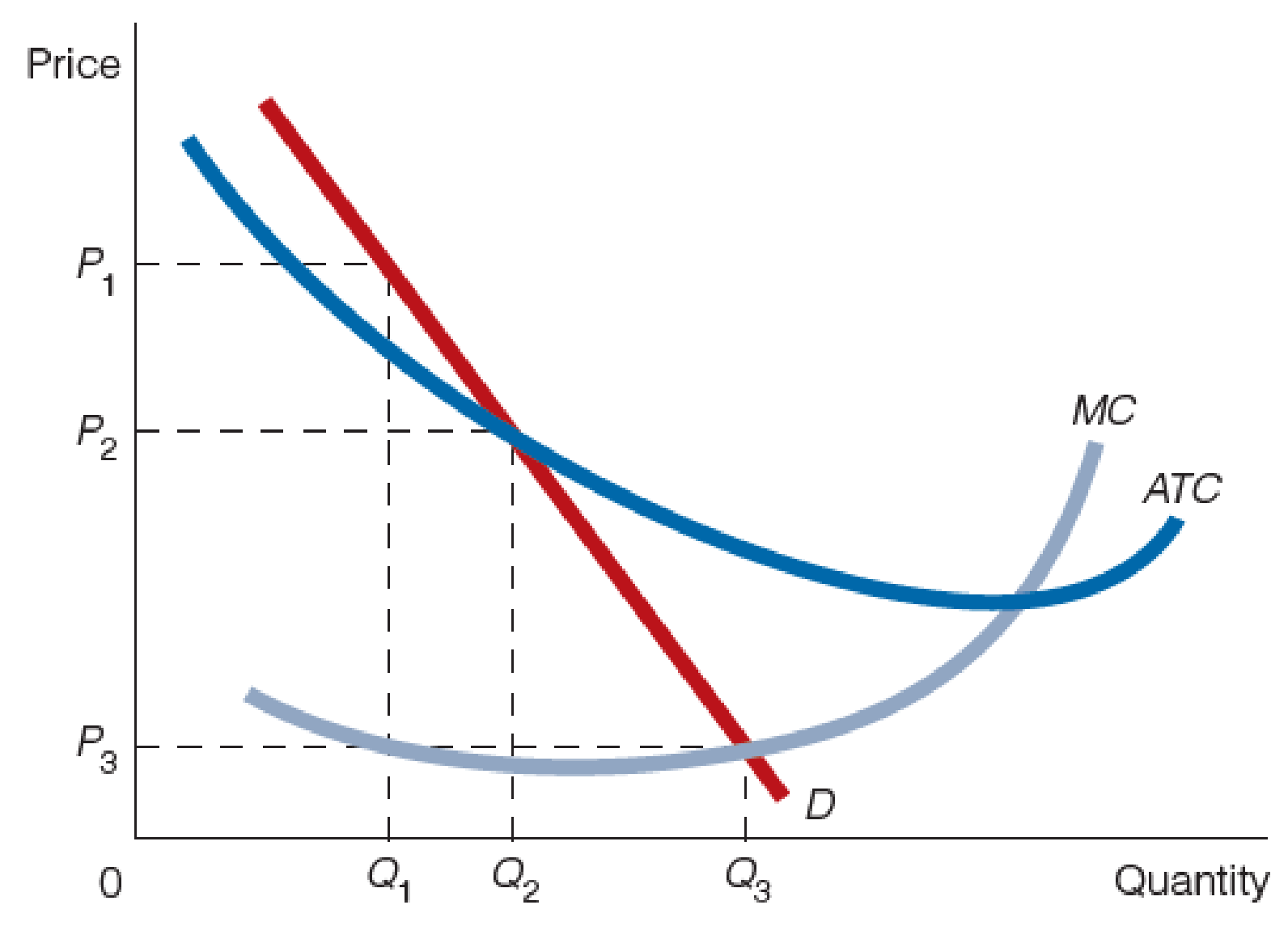Chapter 25, Problem 2WNG

Chapter
Section
Textbook Problem

Use the accompanying figure to answer questions 2–4.2. Is the firm in the figure a natural monopoly? Explain your answer.

To determine

Determine whether the firm is a natural monopoly or not based on the figure.

Explanation

Figure-1 shows the natural monopoly market as follows:

In Figure-1, the horizontal axis measures the quantity and the vertical axis measures the price.

The assumptions of the natural monopoly firm are explained as follows:

• The natural monopoly firm has high fixed costs.
• In the long-run, the average cost curve falls as an increase in the output.
• The socially optimal price-output combination occurs where the price is equal to the marginal cost...

Still sussing out bartleby?

Check out a sample textbook solution.

See a sample solution

The Solution to Your Study Problems

Bartleby provides explanations to thousands of textbook problems written by our experts, many with advanced degrees!

Get Started

Find more solutions based on key concepts